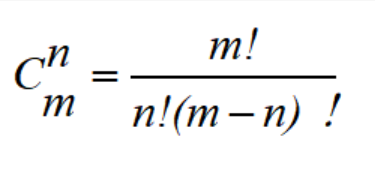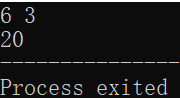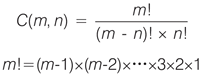• 用c语言编写组合数
2021-11-04 11:32:15

已知组合数的递归定义如下所示，编写递归程序求Ckn

（1）先写递归函数
（2）再写主函数

#include <stdio.h>

int main(){
int seek(int k, int n);
int k, n;
int result;
do{
printf("输入k,n(0<=k<=n):\n");
scanf("%d,%d", &k, &n);
}while(k < 0 || k > n || n < 0);
result = seek(k, n);
printf("%d", result);

}

int seek(int k, int n){
if(k == n || k == 0)
return 1;
return seek(k-1,n-1) + seek(k, n-1);
}

更多相关内容
• ## C语言实现组合数

千次阅读 2021-11-09 15:30:02
组合数公式 //排列组合 #include <stdio.h> int fac(int x) //求阶乘或者说是全排数 { if(x==1) return 1; return x*fac(x-1); } int con(int m,int n) //求组合数 { return fac(m)/(fac(n)*fac...

组合数公式//排列组合
#include <stdio.h>
int fac(int x)			//求阶乘或者说是全排数
{
if(x==1)
return 1;
return x*fac(x-1);
}

int con(int m,int n)	//求组合数
{
return fac(m)/(fac(n)*fac(m-n));
}

int main()
{
int m=0,n=0;		//m>n
scanf("%d %d",&m,&n);
printf("%d",con(m,n));
return 0;
}展开全文组合数
• 这是一个使用C语言函数的示例：计算组合数 C(m,n) 的值(m≤10)。组合数 C(m,n) 可以理解为从 m 个数中任意取出 n 个数的所有情况数。在数学中，求组合数 C(m,n) 的值可以借助 m 和 n 的阶乘来计算，计算公式为：从...

这是一个使用C语言函数的示例：计算组合数 C(m,n) 的值(m≤10)。

组合数 C(m,n) 可以理解为从 m 个数中任意取出 n 个数的所有情况数。在数学中，求组合数 C(m,n) 的值可以借助 m 和 n 的阶乘来计算，计算公式为：从上面的计算公式可以看出，求组合数 C(m,n) 的值，需要进行三次阶乘运算。为了简化程序，可以把阶乘运算设计为函数 fac(x)，求组合数时调用该函数即可。

代码清单 1：计算组合数 C(m,n) 的值(m≤10)

#include

#include

long int fac(int x) //定义阶乘函数

{

int i;

long int s = 1;

for(i=1;i<=x;i++)

s *= i;

return s;

}

int main( ) //主函数

{

int m, n;

printf("输入m和n(m≤10,n≤m):\n");

scanf("%d %d",&m, &n);

printf("C(m,n)=%ld\n",fac(m)/(fac(m-n)*fac(n)));

system("pause");

return 0;

}

运行结果为：

输入m和n(m≤10,n≤m):

11 5

C(m,n)=462

展开全文• 组合数公式 递推求组合数 应用场景： 数据范围小，且有取模条件 // c[a][b] 表示从a中选b个的方案数 for (int i = 0; i < N; i ++ ) for (int j = 0; j <= i; j ++ ) if (!j) c[i][j] = 1; else c...

# 递推求组合数

应用场景：

数据范围小，且有取模条件

// c[a][b] 表示从a中选b个的方案数
for (int i = 0; i < N; i ++ )
for (int j = 0; j <= i; j ++ )
if (!j) c[i][j] = 1;
else c[i][j] = (c[i - 1][j] + c[i - 1][j - 1]) % mod;

# 预处理阶乘求逆元求组合数

应用场景

有取模条件，根据取模条件，如果模数是质数用费马小定理，用快速幂就可求逆元，否则用扩展欧几里得求逆元

费马小定理求逆元

// a 的 k 次方 mod p (p为质数)
int qmi(int a, int k, int p) //快速幂模板
{
int res = 1;
while (k)
{
if (k & 1) res = (long long)res * a % p;
a = (long long)a * a % p;
k >>= 1;
}
return res % p;
}

扩展欧几里得求逆元

// a 为求逆元的数，b 为模数，运算完成后 x 就是在模 a 下的逆元
int exgcd(int a, int b, int &x, int &y)
{
if (!b)
{
x = 1, y = 0;
return a;
}
int d = exgcd(b, a % b, y, x);
y -= a / b * x;
return d;
}

预处理阶乘的逆元

// fact为阶乘 infact为阶乘的逆元
fact = infact = 1;
for (int i = 1; i < N; i ++ )
{
fact[i] = (LL)fact[i - 1] * i % mod;
infact[i] = (LL)infact[i - 1] * qmi(i, mod - 2, mod) % mod;
}

最后运用开头的组合数公式将除法换成逆元乘法并取模就行。

# 卢卡斯定理求组合数

应用场景

求大组合数，a、b > 1e10, and 模数 p 较小的情况下。求逆元根据p的情况来定，为质数可用费马小定理，否则用扩展欧几里得求逆元

根据公式实现

#include<iostream>

using namespace std;
typedef long long LL;
int p;

int ksm(int a, int b)
{
int res = 1;
while (b)
{
if (b & 1)res = (LL)res * a % p;
a = (LL)a * a % p;
b >>= 1;
}
return res;
}

int C(int a, int b)
{
int res = 1;
for (int i = 1, j = a; i <= b; i++, j--)
{
res = (LL)res * j % p;
res = (LL)res * ksm(i, p - 2) % p;
}
return res;
}

int lucas(LL a, LL b)
{
if (a < p && b < p) return C(a, b);
return (LL)C(a % p, b % p) * lucas(a / p, b / p) % p;
}

int main()
{
int n;
cin >> n;
while (n--)
{
LL a, b;
cin >> a >> b >> p;
int res = lucas(a, b);
cout << res << endl;
}

return 0;
}

# 分解质因数求组合数

引用场景

没有取模条件

用公式对a，a - b, b分解质因数消去相同的因子，化简式子用高精度乘法运算解决问题

#include<iostream>
#include<vector>
#include<cmath>

using namespace std;
const int N = 5050;
int primes[N], cnt;//a以内的素数有多少个
bool st[N];
int sum[N];//因子p的k次方，存k
int a, b;

void get_primes(int n)//线性筛
{
for (int i = 2; i <= n; i++)
{
if (!st[i])
{
primes[cnt++] = i;
}
for (int j = 0; primes[j] <= n / i; j++)
{
st[primes[j] * i] = true;
if (i % primes[j] == 0)break;
}
}
}

int get(int a, int p)//get k
{
int sum = 0;
while (a)
{
sum += a / p;
a /= p;
}
return sum;
}

vector<int > mul(vector<int> &a, int b)
{
vector<int> c;
int t = 0;
for (int i = 0; i < a.size() || t; i++)
{
if (i < a.size()) t += a[i] * b;
c.push_back(t % 10);
t /= 10;
}
return c;
}

int main()
{
cin >> a >> b;
//筛素数
get_primes(a);
//
for (int i = 0; i < cnt; i++)
{
int p = primes[i];
//消去相同因子
sum[i] = get(a, p) - get(b, p) - get(a - b, p);
}

vector<int> res;
res.push_back(1);

for (int i = 0; i < cnt ; i++)
{
for (int j = 0; j < sum[i]; j++)
{
res = mul(res, primes[i]);
}
}

for (int i = res.size() - 1; i >= 0; i --) cout << res[i];
cout << endl;

return 0;
}

网格

# 快乐展开全文acm竞赛
• 本题要求编写程序，根据公式算出从n个不同元素中取出m个元素（m≤n）的组合数青少年编程
• 首先输入样例就不对，应该是7 2，... //从n个元素中取m个组合 int n = 0; int m = 0; scanf("%d %d", &n,&m); double ret = fact(n) / (fact(m) * fact(n - m)); printf("%.2f\n", ret); } int fact(i...
• C语言程序设计-编写main程序调用函数fact求解从m个元素选n个元素的组合数的个数;计算公式是： 组合数＝m!(n!.(m-n)!);要求m不能小于n，否则应有容错处理;说明：函数fact(x)的功能是求x!;c语言 开发语言
• #include <stdio.h> int main(int argc, char *argv[]) { printf("%d \n",a(3,8)); printf("%d \n",c(3,8)); return 0; ...//计算排列A(m,n)=n!/m! int a(int m,int n){ ...c/c++
• 计算组合数，利用C语言编程，是最经典的C语言事例
• 解题思路首先要清楚阶乘定义，所谓n的阶乘，就是从1开始乘以比前一个大1的一直乘到n，公式表示就是1×2×3×4×…×(n-2)×(n-1)×n=n!具体步骤（以单个阶乘为例）利用循环，设循环变量为i，初值为1，i从1变化...
• Problem Description In the popular TV series Heroes, there is a tagline "Save the cheerleader, Save the world!". Here Heroes continues, "Solve the puzzle, Save the world!". Finally, alien invaders ...Golang erlang r语言
• 本关任务：编写一个函数实现组合数的计算程序。 编程要求 组合数公式为：C(m,k)=m!/(k!(m-k)!)，请实现求阶乘函数Factorial()和求组合数的函数Combination()，然后从main函数输入m，k,输出组合数。 #include...
• ## C语言实现排列组合

千次阅读 2021-05-20 11:30:25
(1) 全排列：全排列表示把集合中元素的所有按照一定的顺序排列起来，使用P(n, n) = n!表示n个元素全排列的个数。例如：{1, 2, 3}的全排列为：123；132；213；231；312；321；共6个，即3！=321=6。这个是怎么算...
• 已知组合数的递归定义如下所示，编写递归程序求 Cnk 的值（n，k 由键盘输入）。 #include<stdio.h> int main(){ int cnk(int k, int n); int n, k; printf("输入n,k(皆为正整数)"); scanf("%d,%d", &...
• ​算出从n个不同元素中取出m个元素（m≤n）的组合数。 建议定义和调用函数fact(n)计算n!，其中n的类型是int，函数类型是double。 输入格式: 输入在一行中给出两个正整数m和n（m≤n），以空格分隔。 输出格式: ...
• 计算并输出组合数Cmn……不会打……要求递归……谢谢关注:207答案:1mip版解决时间 2021-02-07 18:48提问者彼岸独舞2021-02-06 22:07#includeint A1,A2,A3;int main(){int fac(int n,int m);int n,m,A,k;printf(...
• 要求：将0到9这十个数字分成三个3位数，要求第一个3位数，正好是第二个3位数的1/2，是第三个3位数的1/3。问应当怎样分，编写程序实现。 ...思路：先组合出第一个三位if语句判断每一个数字需
• C语言编写程序求水仙花水仙花是一个数学问题，其实质是一个三位，个位数的立方加十位数的立方加百位数的立方之和等于这个三位本身。例如153＝1*1*1+5*5*5+3*3*3，即153＝1+125+27。这是一个相对较为简单的C...用c语言编写组合数
• 1.编写计算组合数的程序。 问题分析： （1）组合数的计算公式为： （2）此公式中用到3次阶乘的计算，所以可以编写一个求阶层函数fact（x）。 （3）函数fact（x）的功能为计算整数x的阶层。 要求：...
• #include &lt;stdio.h&gt; int main() { int number=123456; int n; for(int i=2;i&gt;=0;i--){ scanf("%d",&amp;n); if(number==n){ ...输入错误，你还有%d次...
• C语言 代码总行：478行 函数总数：16个（包括main函数） 初版时间：2017年3月5日 此版时间：2021年4月23日 3、核心算法与象棋规则、术语 3.1 行棋规则与行棋判定函数设计 在这里，只考虑了红方棋子的情况，目的是...编程语言
• 任意一个每位数字不完全相同的 4 位正整数，将各位上数字重新组合成一个最大和一个最小相减，重复这个过程，必定得到 6174. 输入描述 有多组测试数据，每组测试数据占一行. 每行输入一个 44 位正整数. 输出描述 ...数据结构
• 在当今,流行的编程软件种类繁多，它们编程方便、易于维护，但是在与硬件直接打交道和编制系统软件时却束手无策，于是C语言就有了用武之地。C语言作为汇编语言与高级语言之间的一种过渡语言，兼有汇编语言的高效和...
• 1100: 求组合数（函数专题） 时间限制: 1 Sec 内存限制: 128 MB 提交: 16333 解决: 11121 [状态] [讨论版] [提交] [命题人:admin] 题目描述 马上要举办新生程序设计竞赛了，与以往不同的是，本次比赛以班为单位，...
• 编写函数求组合数C= n! / (k! *( n-k)!) 程序流程图： 代码： #include<stdio.h> int mul(int x,int y); void main() { int n,k; double c; printf("please enter n:\tk:\t"); scanf("%d,%d",&n,&k);...
• 1.编写计算组合数的程序。 问题分析： （1）组合数的计算公式为： （2）此公式中用到3次阶乘的计算，所以可以编写一个求阶层函数fact（x）。 （3）函数fact（x）的功能为计算整数x的阶层。 要求...
• c语言编程问题,计算出从n 个不同元素中取出m 个元素(m≤n)的组合数编写程序2018-3-8来自ip:18.113.162.140的网友咨询浏览量:374问题补充：c语言编程问题,计算出从n 个不同元素中取出m 个元素(m≤n)的组合数编写...
• 4 补充说明 虽然 KLKMN 只提供了 语言编写用户自定义函数的一 个模板 但设置 函数的不同的返回值和主参数 模板中提供了 各种组合 详见 FEA4 完全可以开发出功能强大的自定义函 来丰富 KLKMN OFIP 的函数库 来...
• 原标题：C语言邮票组合代码解析我们寄信都要贴邮票，在邮局有一些小面值的邮票，通过这些小面值邮票中的一张或几张的组合，可以满足不同邮件的不同邮资。现在，邮局有4种不同面值的邮票。在每个信封上最多能贴5张...
• 排列有两种定义，但计算方法只有一种，凡是符合这两种定义的都这种方法计算。定义的前提条件是m≦n，m与n均为自然数。下面介绍排列组合c的计算方法及公式，供参考。排列组合中A和C怎么算排列A(n,m)=n×(n-1).(n-m+...c语言中组合函数...